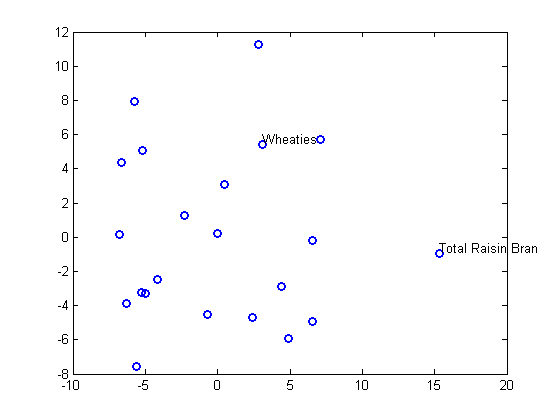## Nonclassical and Nonmetric Multidimensional Scaling

Perform nonclassical multidimensional scaling using `mdscale`.

### Nonclassical Multidimensional Scaling

The function `mdscale` performs nonclassical multidimensional scaling. As with `cmdscale`, you use `mdscale` either to visualize dissimilarity data for which no “locations” exist, or to visualize high-dimensional data by reducing its dimensionality. Both functions take a matrix of dissimilarities as an input and produce a configuration of points. However, `mdscale` offers a choice of different criteria to construct the configuration, and allows missing data and weights.

For example, the cereal data include measurements on 10 variables describing breakfast cereals. You can use `mdscale` to visualize these data in two dimensions. First, load the data. For clarity, this example code selects a subset of 22 of the observations.

```load cereal.mat X = [Calories Protein Fat Sodium Fiber ... Carbo Sugars Shelf Potass Vitamins]; % Take a subset from a single manufacturer mfg1 = strcmp('G',cellstr(Mfg)); X = X(mfg1,:); size(X) ans = 22 10```

Then use `pdist` to transform the 10-dimensional data into dissimilarities. The output from `pdist` is a symmetric dissimilarity matrix, stored as a vector containing only the (23*22/2) elements in its upper triangle.

```dissimilarities = pdist(zscore(X),'cityblock'); size(dissimilarities) ans = 1 231```

This example code first standardizes the cereal data, and then uses city block distance as a dissimilarity. The choice of transformation to dissimilarities is application-dependent, and the choice here is only for simplicity. In some applications, the original data are already in the form of dissimilarities.

Next, use `mdscale` to perform metric MDS. Unlike `cmdscale`, you must specify the desired number of dimensions, and the method to use to construct the output configuration. For this example, use two dimensions. The metric STRESS criterion is a common method for computing the output; for other choices, see the `mdscale` reference page in the online documentation. The second output from `mdscale` is the value of that criterion evaluated for the output configuration. It measures the how well the inter-point distances of the output configuration approximate the original input dissimilarities:

```[Y,stress] =... mdscale(dissimilarities,2,'criterion','metricstress'); stress stress = 0.1856```

A scatterplot of the output from `mdscale` represents the original 10-dimensional data in two dimensions, and you can use the `gname` function to label selected points:

```plot(Y(:,1),Y(:,2),'o','LineWidth',2); gname(Name(mfg1))```### Nonmetric Multidimensional Scaling

Metric multidimensional scaling creates a configuration of points whose inter-point distances approximate the given dissimilarities. This is sometimes too strict a requirement, and non-metric scaling is designed to relax it a bit. Instead of trying to approximate the dissimilarities themselves, non-metric scaling approximates a nonlinear, but monotonic, transformation of them. Because of the monotonicity, larger or smaller distances on a plot of the output will correspond to larger or smaller dissimilarities, respectively. However, the nonlinearity implies that `mdscale` only attempts to preserve the ordering of dissimilarities. Thus, there may be contractions or expansions of distances at different scales.

You use `mdscale` to perform nonmetric MDS in much the same way as for metric scaling. The nonmetric STRESS criterion is a common method for computing the output; for more choices, see the `mdscale` reference page in the online documentation. As with metric scaling, the second output from `mdscale` is the value of that criterion evaluated for the output configuration. For nonmetric scaling, however, it measures the how well the inter-point distances of the output configuration approximate the disparities. The disparities are returned in the third output. They are the transformed values of the original dissimilarities:

```[Y,stress,disparities] = ... mdscale(dissimilarities,2,'criterion','stress'); stress stress = 0.1562```

To check the fit of the output configuration to the dissimilarities, and to understand the disparities, it helps to make a Shepard plot:

```distances = pdist(Y); [dum,ord] = sortrows([disparities(:) dissimilarities(:)]); plot(dissimilarities,distances,'bo', ... dissimilarities(ord),disparities(ord),'r.-', ... [0 25],[0 25],'k-') xlabel('Dissimilarities') ylabel('Distances/Disparities') legend({'Distances' 'Disparities' '1:1 Line'},... 'Location','NorthWest');```This plot shows that `mdscale` has found a configuration of points in two dimensions whose inter-point distances approximates the disparities, which in turn are a nonlinear transformation of the original dissimilarities. The concave shape of the disparities as a function of the dissimilarities indicates that fit tends to contract small distances relative to the corresponding dissimilarities. This may be perfectly acceptable in practice.

`mdscale` uses an iterative algorithm to find the output configuration, and the results can often depend on the starting point. By default, `mdscale` uses `cmdscale` to construct an initial configuration, and this choice often leads to a globally best solution. However, it is possible for `mdscale` to stop at a configuration that is a local minimum of the criterion. Such cases can be diagnosed and often overcome by running `mdscale` multiple times with different starting points. You can do this using the `'start'` and `'replicates'` name-value pair arguments. The following code runs five replicates of MDS, each starting at a different randomly-chosen initial configuration. The criterion value is printed out for each replication; `mdscale` returns the configuration with the best fit.

```opts = statset('Display','final'); [Y,stress] =... mdscale(dissimilarities,2,'criterion','stress',... 'start','random','replicates',5,'Options',opts); 35 iterations, Final stress criterion = 0.156209 31 iterations, Final stress criterion = 0.156209 48 iterations, Final stress criterion = 0.171209 33 iterations, Final stress criterion = 0.175341 32 iterations, Final stress criterion = 0.185881```

Notice that `mdscale` finds several different local solutions, some of which do not have as low a stress value as the solution found with the `cmdscale` starting point.